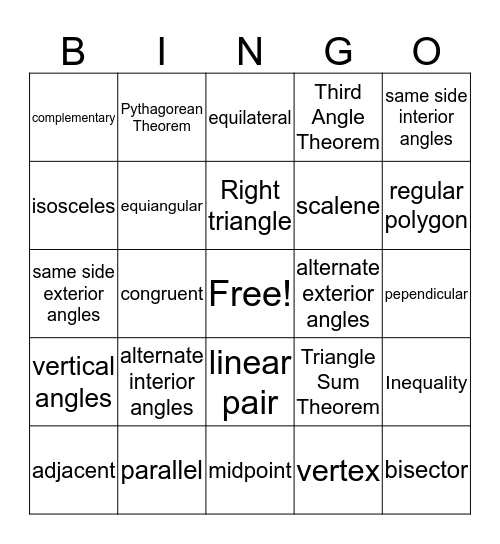Geometry BingoThis bingo card has a free space and 29 words: adjacent, transversal, congruent, supplementary, complementary, equilateral, equiangular, alternate interior angles, alternate exterior angles, parallel, pependicular, bisector, midpoint, vertex, regular polygon, corresponding angles, linear pair, vertical angles, same side interior angles, same side exterior angles, Triangle Sum Theorem, Exterior Angle Theorem, scalene, isosceles, Third Angle Theorem, Pythagorean Theorem, Inequality, Right triangle and Distance Formula.

Play Online